Anúncio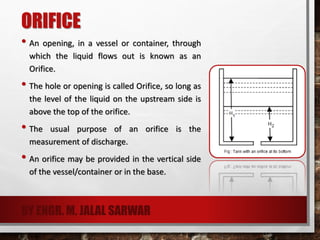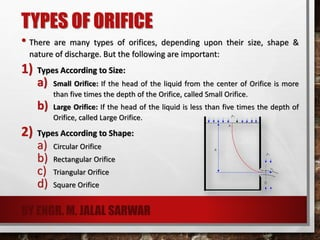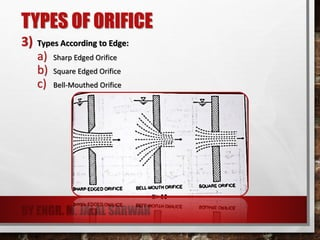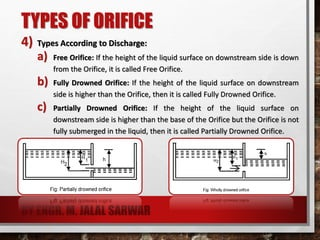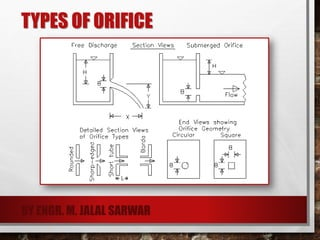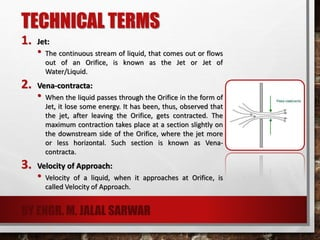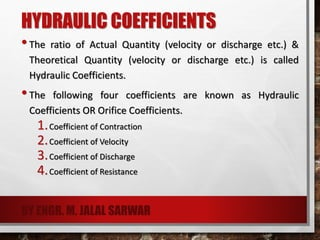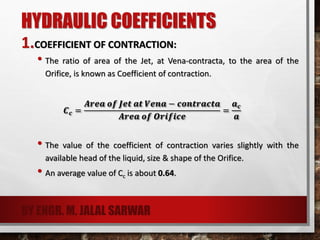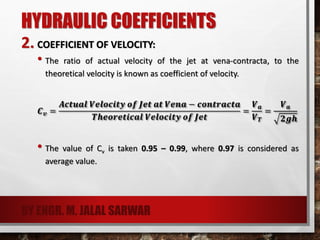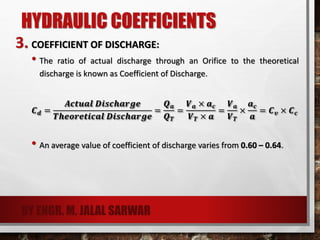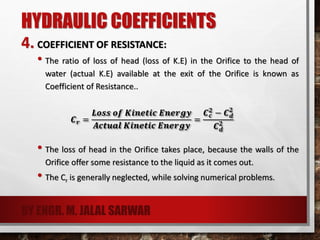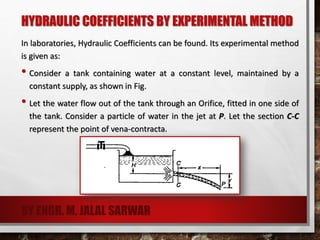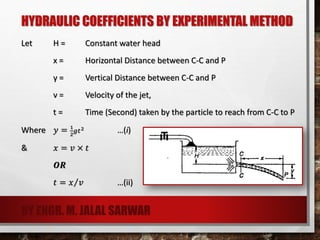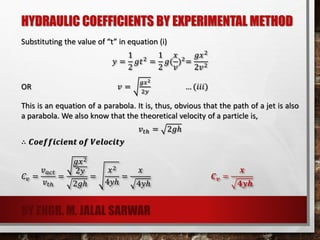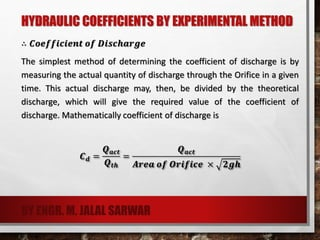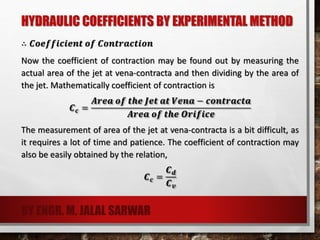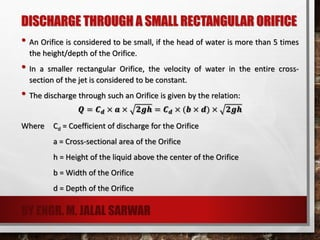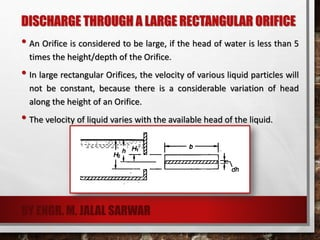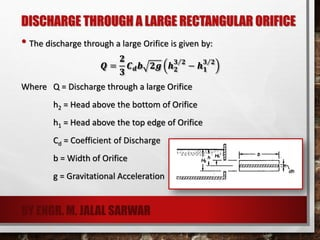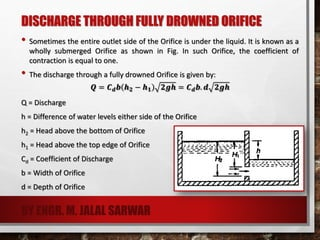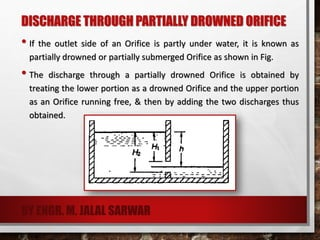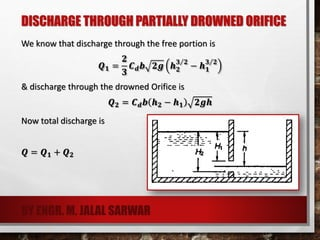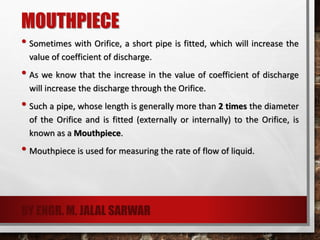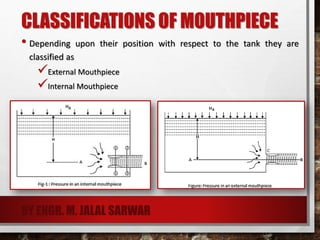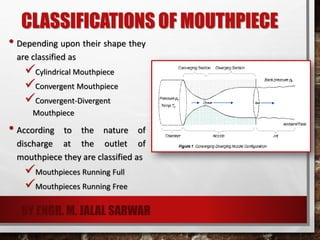1 de 27
Anúncio

### Flow Through Orifices - Hydraulics

1. ORIFICE • An opening, in a vessel or container, through which the liquid flows out is known as an Orifice. • The hole or opening is called Orifice, so long as the level of the liquid on the upstream side is above the top of the orifice. • The usual purpose of an orifice is the measurement of discharge. • An orifice may be provided in the vertical side of the vessel/container or in the base. BY ENGR. M. JALAL SARWAR
2. TYPES OF ORIFICE • There are many types of orifices, depending upon their size, shape & nature of discharge. But the following are important: 1) Types According to Size: a) Small Orifice: If the head of the liquid from the center of Orifice is more than five times the depth of the Orifice, called Small Orifice. b) Large Orifice: If the head of the liquid is less than five times the depth of Orifice, called Large Orifice. 2) Types According to Shape: a) Circular Orifice b) Rectangular Orifice c) Triangular Orifice d) Square Orifice BY ENGR. M. JALAL SARWAR
3. TYPES OF ORIFICE 3) Types According to Edge: a) Sharp Edged Orifice b) Square Edged Orifice c) Bell-Mouthed Orifice BY ENGR. M. JALAL SARWAR
4. TYPES OF ORIFICE 4) Types According to Discharge: a) Free Orifice: If the height of the liquid surface on downstream side is down from the Orifice, it is called Free Orifice. b) Fully Drowned Orifice: If the height of the liquid surface on downstream side is higher than the Orifice, then it is called Fully Drowned Orifice. c) Partially Drowned Orifice: If the height of the liquid surface on downstream side is higher than the base of the Orifice but the Orifice is not fully submerged in the liquid, then it is called Partially Drowned Orifice. BY ENGR. M. JALAL SARWAR
5. TYPES OF ORIFICE BY ENGR. M. JALAL SARWAR
6. TECHNICAL TERMS 1. Jet: • The continuous stream of liquid, that comes out or flows out of an Orifice, is known as the Jet or Jet of Water/Liquid. 2. Vena-contracta: • When the liquid passes through the Orifice in the form of Jet, it lose some energy. It has been, thus, observed that the jet, after leaving the Orifice, gets contracted. The maximum contraction takes place at a section slightly on the downstream side of the Orifice, where the jet more or less horizontal. Such section is known as Vena- contracta. 3. Velocity of Approach: • Velocity of a liquid, when it approaches at Orifice, is called Velocity of Approach. BY ENGR. M. JALAL SARWAR
7. HYDRAULIC COEFFICIENTS •The ratio of Actual Quantity (velocity or discharge etc.) & Theoretical Quantity (velocity or discharge etc.) is called Hydraulic Coefficients. •The following four coefficients are known as Hydraulic Coefficients OR Orifice Coefficients. 1.Coefficient of Contraction 2.Coefficient of Velocity 3.Coefficient of Discharge 4.Coefficient of Resistance BY ENGR. M. JALAL SARWAR
8. HYDRAULIC COEFFICIENTS 1.COEFFICIENT OF CONTRACTION: • The ratio of area of the Jet, at Vena-contracta, to the area of the Orifice, is known as Coefficient of contraction. 𝑪 𝒄 = 𝑨𝒓𝒆𝒂 𝒐𝒇 𝑱𝒆𝒕 𝒂𝒕 𝑽𝒆𝒏𝒂 − 𝒄𝒐𝒏𝒕𝒓𝒂𝒄𝒕𝒂 𝑨𝒓𝒆𝒂 𝒐𝒇 𝑶𝒓𝒊𝒇𝒊𝒄𝒆 = 𝒂 𝒄 𝒂 • The value of the coefficient of contraction varies slightly with the available head of the liquid, size & shape of the Orifice. • An average value of Cc is about 0.64. BY ENGR. M. JALAL SARWAR
9. HYDRAULIC COEFFICIENTS 2. COEFFICIENT OF VELOCITY: • The ratio of actual velocity of the jet at vena-contracta, to the theoretical velocity is known as coefficient of velocity. 𝑪 𝒗 = 𝑨𝒄𝒕𝒖𝒂𝒍 𝑽𝒆𝒍𝒐𝒄𝒊𝒕𝒚 𝒐𝒇 𝑱𝒆𝒕 𝒂𝒕 𝑽𝒆𝒏𝒂 − 𝒄𝒐𝒏𝒕𝒓𝒂𝒄𝒕𝒂 𝑻𝒉𝒆𝒐𝒓𝒆𝒕𝒊𝒄𝒂𝒍 𝑽𝒆𝒍𝒐𝒄𝒊𝒕𝒚 𝒐𝒇 𝑱𝒆𝒕 = 𝑽 𝒂 𝑽 𝑻 = 𝑽 𝒂 𝟐𝒈𝒉 • The value of Cv is taken 0.95 – 0.99, where 0.97 is considered as average value. BY ENGR. M. JALAL SARWAR
10. HYDRAULIC COEFFICIENTS 3. COEFFICIENT OF DISCHARGE: • The ratio of actual discharge through an Orifice to the theoretical discharge is known as Coefficient of Discharge. 𝑪 𝒅 = 𝑨𝒄𝒕𝒖𝒂𝒍 𝑫𝒊𝒔𝒄𝒉𝒂𝒓𝒈𝒆 𝑻𝒉𝒆𝒐𝒓𝒆𝒕𝒊𝒄𝒂𝒍 𝑫𝒊𝒔𝒄𝒉𝒂𝒓𝒈𝒆 = 𝑸 𝒂 𝑸 𝑻 = 𝑽 𝒂 × 𝒂 𝒄 𝑽 𝑻 × 𝒂 = 𝑽 𝒂 𝑽 𝑻 × 𝒂 𝒄 𝒂 = 𝑪 𝒗 × 𝑪 𝒄 • An average value of coefficient of discharge varies from 0.60 – 0.64. BY ENGR. M. JALAL SARWAR
11. HYDRAULIC COEFFICIENTS 4. COEFFICIENT OF RESISTANCE: • The ratio of loss of head (loss of K.E) in the Orifice to the head of water (actual K.E) available at the exit of the Orifice is known as Coefficient of Resistance.. 𝑪 𝒓 = 𝑳𝒐𝒔𝒔 𝒐𝒇 𝑲𝒊𝒏𝒆𝒕𝒊𝒄 𝑬𝒏𝒆𝒓𝒈𝒚 𝑨𝒄𝒕𝒖𝒂𝒍 𝑲𝒊𝒏𝒆𝒕𝒊𝒄 𝑬𝒏𝒆𝒓𝒈𝒚 = 𝑪 𝒄 𝟐 − 𝑪 𝒅 𝟐 𝑪 𝒅 𝟐 • The loss of head in the Orifice takes place, because the walls of the Orifice offer some resistance to the liquid as it comes out. • The Cr is generally neglected, while solving numerical problems. BY ENGR. M. JALAL SARWAR
12. HYDRAULIC COEFFICIENTS BY EXPERIMENTAL METHOD In laboratories, Hydraulic Coefficients can be found. Its experimental method is given as: • Consider a tank containing water at a constant level, maintained by a constant supply, as shown in Fig. • Let the water flow out of the tank through an Orifice, fitted in one side of the tank. Consider a particle of water in the jet at P. Let the section C-C represent the point of vena-contracta. BY ENGR. M. JALAL SARWAR
13. HYDRAULIC COEFFICIENTS BY EXPERIMENTAL METHOD Let H = Constant water head x = Horizontal Distance between C-C and P y = Vertical Distance between C-C and P v = Velocity of the jet, t = Time (Second) taken by the particle to reach from C-C to P Where 𝑦 = 1 2 𝑔𝑡2 …(i) & 𝑥 = 𝑣 × 𝑡 𝑶𝑹 𝑡 = 𝑥 𝑣 …(ii) BY ENGR. M. JALAL SARWAR
14. HYDRAULIC COEFFICIENTS BY EXPERIMENTAL METHOD Substituting the value of “t” in equation (i) 𝑦 = 1 2 𝑔𝑡2 = 1 2 𝑔( 𝑥 𝑣 )2 = 𝑔𝑥2 2𝑣2 OR 𝑣 = 𝑔𝑥2 2𝑦 … (𝑖𝑖𝑖) This is an equation of a parabola. It is, thus, obvious that the path of a jet is also a parabola. We also know that the theoretical velocity of a particle is, 𝑣𝑡ℎ = 2𝑔ℎ ∴ 𝑪𝒐𝒆𝒇𝒇𝒊𝒄𝒊𝒆𝒏𝒕 𝒐𝒇 𝑽𝒆𝒍𝒐𝒄𝒊𝒕𝒚 𝐶𝑣 = 𝑣 𝑎𝑐𝑡 𝑣𝑡ℎ = 𝑔𝑥2 2𝑦 2𝑔ℎ = 𝑥2 4𝑦ℎ = 𝑥 4𝑦ℎ 𝑪 𝒗 = 𝒙 𝟒𝒚𝒉 BY ENGR. M. JALAL SARWAR
15. HYDRAULIC COEFFICIENTS BY EXPERIMENTAL METHOD ∴ 𝑪𝒐𝒆𝒇𝒇𝒊𝒄𝒊𝒆𝒏𝒕 𝒐𝒇 𝑫𝒊𝒔𝒄𝒉𝒂𝒓𝒈𝒆 The simplest method of determining the coefficient of discharge is by measuring the actual quantity of discharge through the Orifice in a given time. This actual discharge may, then, be divided by the theoretical discharge, which will give the required value of the coefficient of discharge. Mathematically coefficient of discharge is 𝑪 𝒅 = 𝑸 𝒂𝒄𝒕 𝑸 𝒕𝒉 = 𝑸 𝒂𝒄𝒕 𝑨𝒓𝒆𝒂 𝒐𝒇 𝑶𝒓𝒊𝒇𝒊𝒄𝒆 × 𝟐𝒈𝒉 BY ENGR. M. JALAL SARWAR
16. HYDRAULIC COEFFICIENTS BY EXPERIMENTAL METHOD ∴ 𝑪𝒐𝒆𝒇𝒇𝒊𝒄𝒊𝒆𝒏𝒕 𝒐𝒇 𝑪𝒐𝒏𝒕𝒓𝒂𝒄𝒕𝒊𝒐𝒏 Now the coefficient of contraction may be found out by measuring the actual area of the jet at vena-contracta and then dividing by the area of the jet. Mathematically coefficient of contraction is 𝑪 𝒄 = 𝑨𝒓𝒆𝒂 𝒐𝒇 𝒕𝒉𝒆 𝑱𝒆𝒕 𝒂𝒕 𝑽𝒆𝒏𝒂 − 𝒄𝒐𝒏𝒕𝒓𝒂𝒄𝒕𝒂 𝑨𝒓𝒆𝒂 𝒐𝒇 𝒕𝒉𝒆 𝑶𝒓𝒊𝒇𝒊𝒄𝒆 The measurement of area of the jet at vena-contracta is a bit difficult, as it requires a lot of time and patience. The coefficient of contraction may also be easily obtained by the relation, 𝑪 𝒄 = 𝑪 𝒅 𝑪 𝒗 BY ENGR. M. JALAL SARWAR
17. DISCHARGE THROUGH A SMALL RECTANGULAR ORIFICE • An Orifice is considered to be small, if the head of water is more than 5 times the height/depth of the Orifice. • In a smaller rectangular Orifice, the velocity of water in the entire cross- section of the jet is considered to be constant. • The discharge through such an Orifice is given by the relation: 𝑸 = 𝑪 𝒅 × 𝒂 × 𝟐𝒈𝒉 = 𝑪 𝒅 × (𝒃 × 𝒅) × 𝟐𝒈𝒉 Where Cd = Coefficient of discharge for the Orifice a = Cross-sectional area of the Orifice h = Height of the liquid above the center of the Orifice b = Width of the Orifice d = Depth of the Orifice BY ENGR. M. JALAL SARWAR
18. DISCHARGE THROUGH A LARGE RECTANGULAR ORIFICE • An Orifice is considered to be large, if the head of water is less than 5 times the height/depth of the Orifice. • In large rectangular Orifices, the velocity of various liquid particles will not be constant, because there is a considerable variation of head along the height of an Orifice. • The velocity of liquid varies with the available head of the liquid. BY ENGR. M. JALAL SARWAR
19. DISCHARGE THROUGH A LARGE RECTANGULAR ORIFICE • The discharge through a large Orifice is given by: 𝑸 = 𝟐 𝟑 𝑪 𝒅 𝒃 𝟐𝒈 𝒉 𝟐 𝟑 𝟐 − 𝒉 𝟏 𝟑 𝟐 Where Q = Discharge through a large Orifice h2 = Head above the bottom of Orifice h1 = Head above the top edge of Orifice Cd = Coefficient of Discharge b = Width of Orifice g = Gravitational Acceleration BY ENGR. M. JALAL SARWAR
20. DISCHARGE THROUGH FULLY DROWNED ORIFICE • Sometimes the entire outlet side of the Orifice is under the liquid. It is known as a wholly submerged Orifice as shown in Fig. In such Orifice, the coefficient of contraction is equal to one. • The discharge through a fully drowned Orifice is given by: 𝑸 = 𝑪 𝒅 𝒃 𝒉 𝟐 − 𝒉 𝟏 𝟐𝒈𝒉 = 𝑪 𝒅 𝒃. 𝒅 𝟐𝒈𝒉 Q = Discharge h = Difference of water levels either side of the Orifice h2 = Head above the bottom of Orifice h1 = Head above the top edge of Orifice Cd = Coefficient of Discharge b = Width of Orifice d = Depth of Orifice BY ENGR. M. JALAL SARWAR
21. DISCHARGE THROUGH PARTIALLY DROWNED ORIFICE • If the outlet side of an Orifice is partly under water, it is known as partially drowned or partially submerged Orifice as shown in Fig. • The discharge through a partially drowned Orifice is obtained by treating the lower portion as a drowned Orifice and the upper portion as an Orifice running free, & then by adding the two discharges thus obtained. BY ENGR. M. JALAL SARWAR
22. DISCHARGE THROUGH PARTIALLY DROWNED ORIFICE We know that discharge through the free portion is 𝑸 𝟏 = 𝟐 𝟑 𝑪 𝒅 𝒃 𝟐𝒈 𝒉 𝟐 𝟑 𝟐 − 𝒉 𝟏 𝟑 𝟐 & discharge through the drowned Orifice is 𝑸 𝟐 = 𝑪 𝒅 𝒃 𝒉 𝟐 − 𝒉 𝟏 𝟐𝒈𝒉 Now total discharge is 𝑸 = 𝑸 𝟏 + 𝑸 𝟐 BY ENGR. M. JALAL SARWAR
23. MOUTHPIECE • Sometimes with Orifice, a short pipe is fitted, which will increase the value of coefficient of discharge. • As we know that the increase in the value of coefficient of discharge will increase the discharge through the Orifice. • Such a pipe, whose length is generally more than 2 times the diameter of the Orifice and is fitted (externally or internally) to the Orifice, is known as a Mouthpiece. • Mouthpiece is used for measuring the rate of flow of liquid. BY ENGR. M. JALAL SARWAR
24. CLASSIFICATIONS OF MOUTHPIECE • Depending upon their position with respect to the tank they are classified as External Mouthpiece Internal Mouthpiece BY ENGR. M. JALAL SARWAR
25. CLASSIFICATIONS OF MOUTHPIECE • Depending upon their shape they are classified as Cylindrical Mouthpiece Convergent Mouthpiece Convergent-Divergent Mouthpiece • According to the nature of discharge at the outlet of mouthpiece they are classified as Mouthpieces Running Full Mouthpieces Running Free BY ENGR. M. JALAL SARWAR
Anúncio